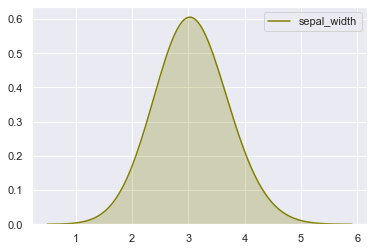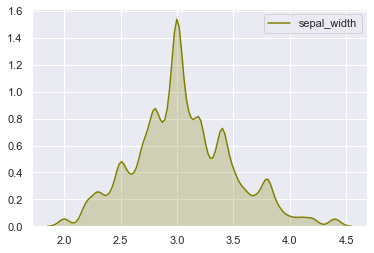The following density plots have been made using the same data. Only the bandwidth value changes from 0.5 in the first graph to 0.05 on the right. This is controlled using the bw argument of the kdeplot function (seaborn library).

This parameter can be of particular interest when a finer understanding of the distribution is needed. It could highlight bimodal distributions more easily and help us in observing patterns that the Gaussian kernel over-smoothed.

Note that 'bw' parameter is deprecated since seaborn 0.11.0 and 'bw_method' and 'bw_value' are to be used. See scipy.stats.gaussian_kde in scipy.org for further details on bw_method and bw_value.

``````# libraries & dataset
import seaborn as sns
import matplotlib.pyplot as plt
# set a grey background (use sns.set_theme() if seaborn version 0.11.0 or above)
sns.set(style="darkgrid")

# Large bandwidth
plt.show()``````In seaborn 0.11.0 and later versions, you would use sns.kdeplot(df['sepal_width'], shade=True, bw_method=0.05, color='olive')

``````# libraries & dataset
import seaborn as sns
import matplotlib.pyplot as plt
# set a grey background (use sns.set_theme() if seaborn version 0.11.0 or above)
sns.set(style="darkgrid")